Indev 1.2

Biggest updates: Animation, Object-placer, object-selecter, gui.

Posted by on

In this version I added some new core features.

GUI:
It looks horrible but it works for now.
You can already use the buttons on the top to change the playspeed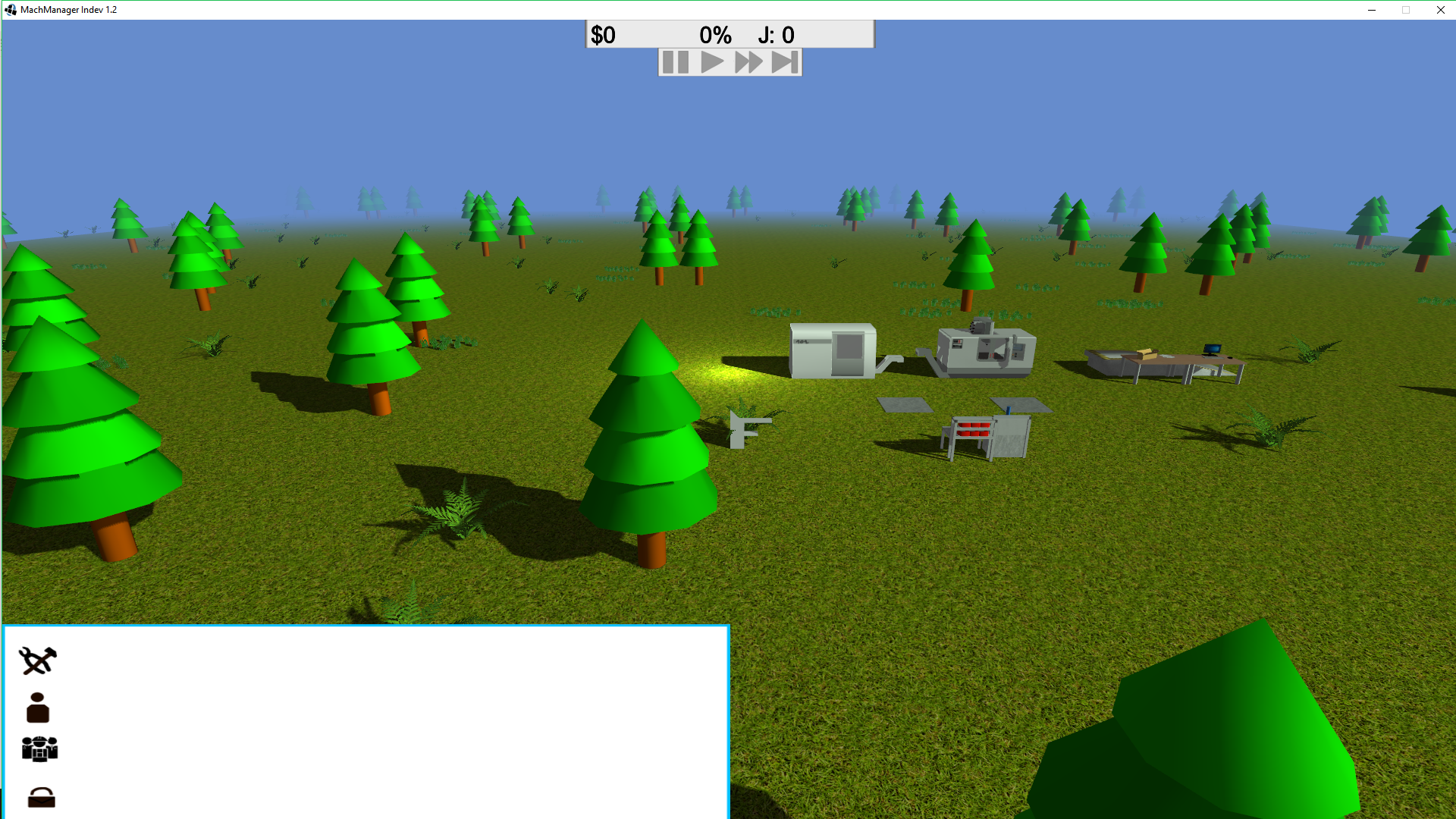Object-placer:
With that you can place things on the floor. It works with a ray that is cast from the mouse position on the camera wich get checked if it hits the floor.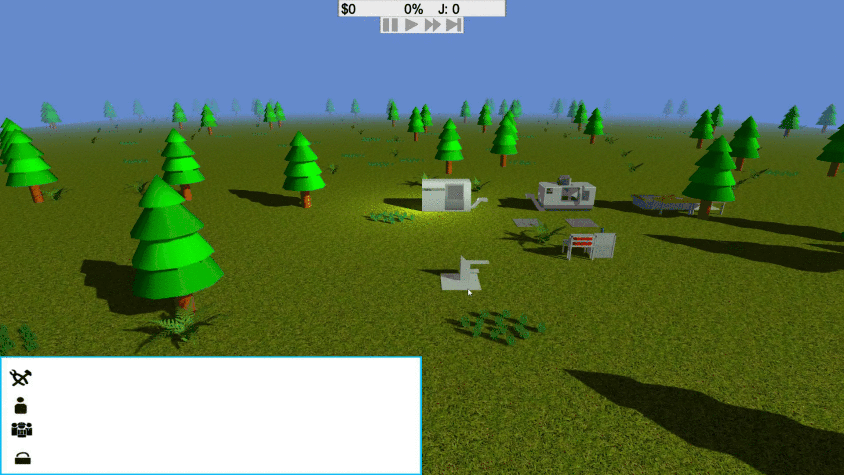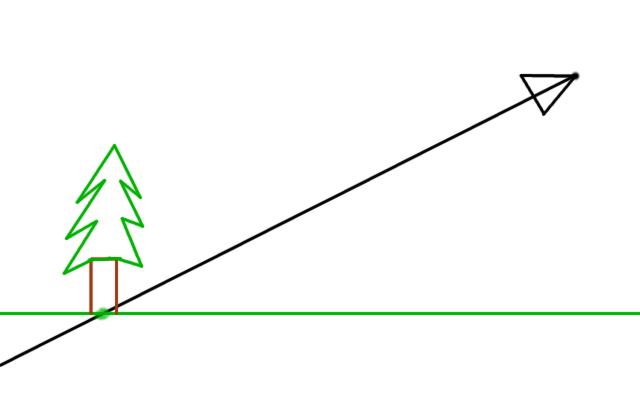Object-selecter:
Works with someting called AABB collistion detection. Wich uses the same ray as above to check if the ray is inside a defined box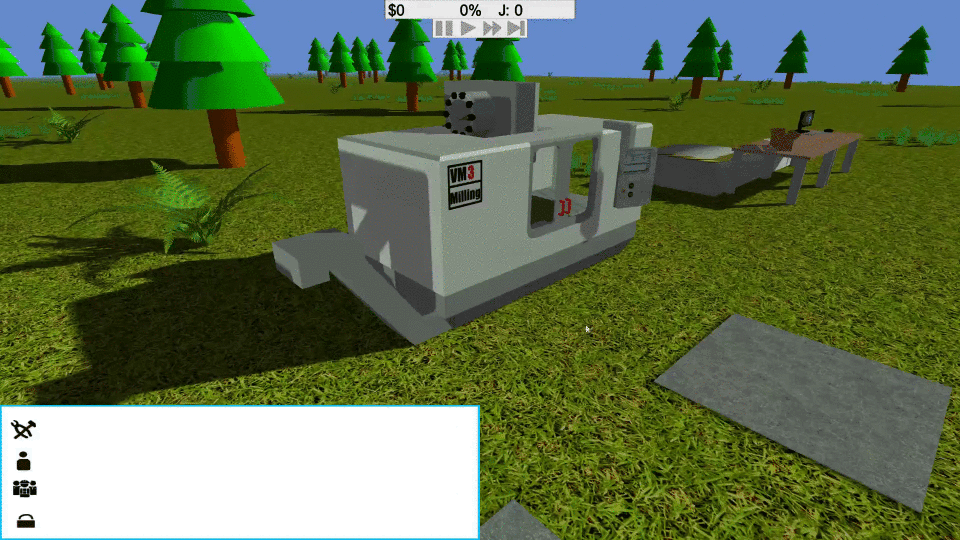There is the code for it:

``````public static boolean calcselect(Vector3f lb, Vector3f rt){
float t1 = (lb.x - camera.getPosition().x)*inverseRay.x;
float t2 = (rt.x - camera.getPosition().x)*inverseRay.x;
float t3 = (lb.y - camera.getPosition().y)*inverseRay.y;
float t4 = (rt.y - camera.getPosition().y)*inverseRay.y;
float t5 = (lb.z - camera.getPosition().z)*inverseRay.z;
float t6 = (rt.z - camera.getPosition().z)*inverseRay.z;

float tmin = Math.max(Math.max(Math.min(t1, t2), Math.min(t3, t4)), Math.min(t5, t6));
float tmax = Math.min(Math.min(Math.max(t1, t2), Math.max(t3, t4)), Math.max(t5, t6));

if(tmax < 0)return false;
if(tmin > tmax)return false;
return true;
}``````

Animation:
Animation was the biggest and hardest topic in this version.
I used the code from ThinMatrix as a base wich was already pretty complicated.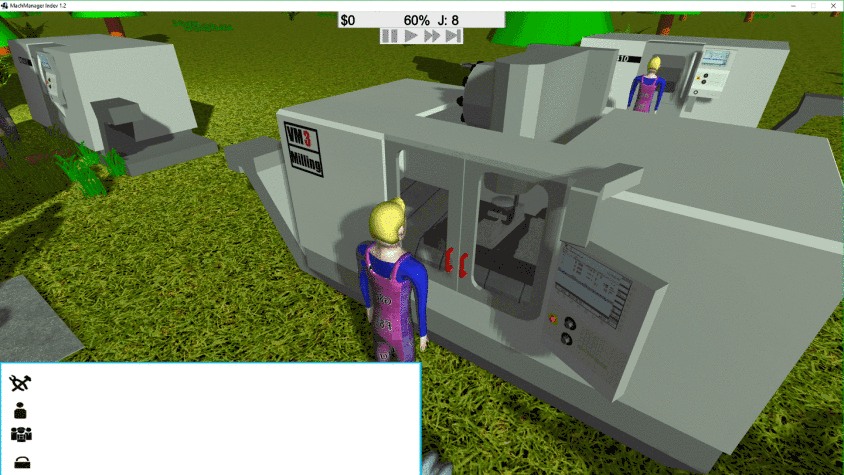Ohers:
There are some other things going on.
- Logistic network has been rewritten and works now bugfree.
- Some redoing in the Entity-loader system.
- Workers are now rotating in the direction in wich they move.
- Some minor bugfixing

New Model: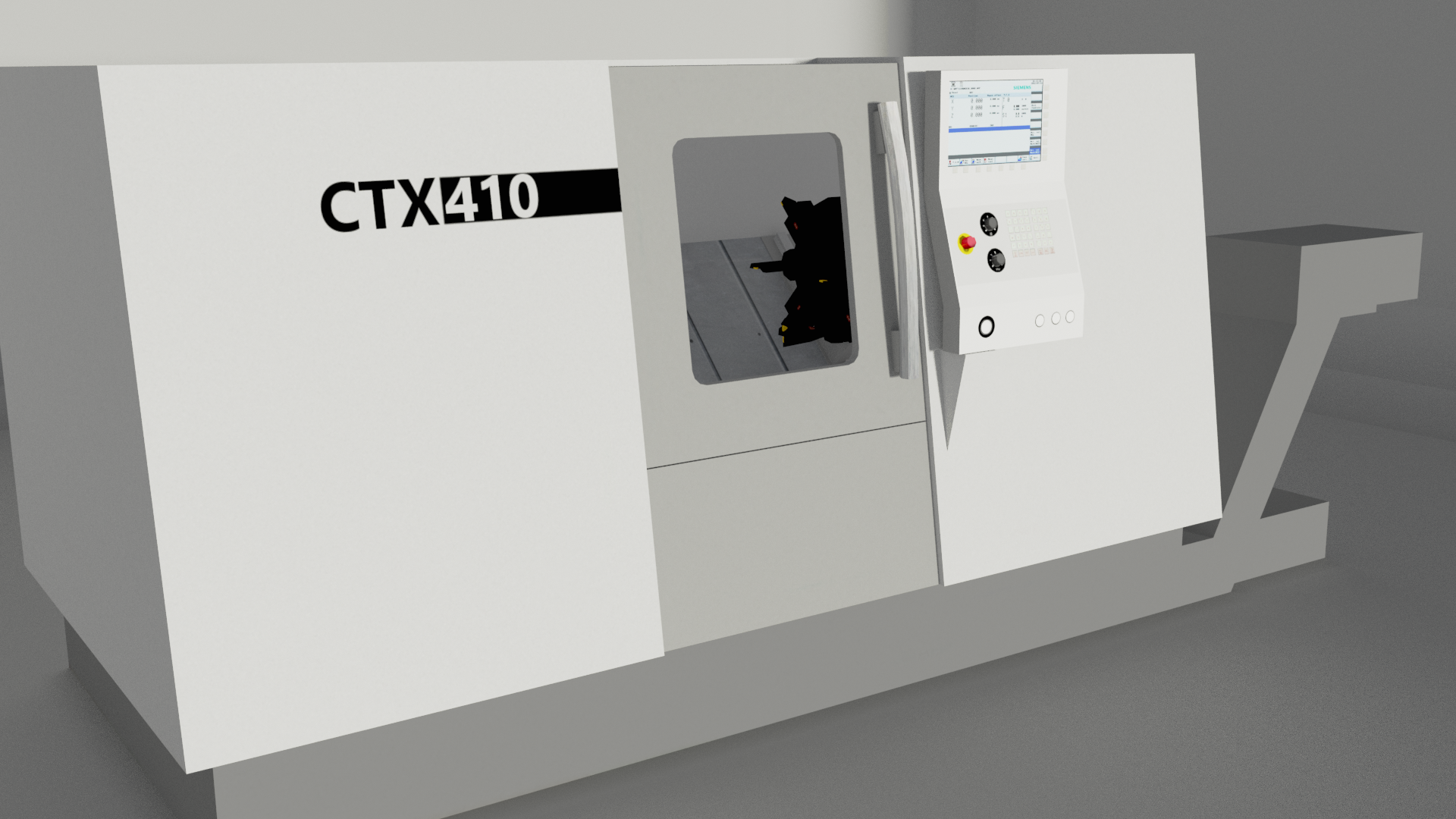Indev 1.3 Topics:
- Rooms
- AStar pathfinder
- Welding and sawing machines
- Machines spare jobs
- frustum culling
- some bugfixing and refactoring